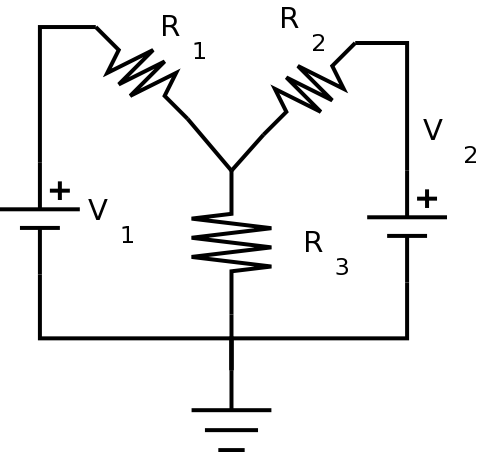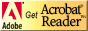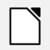# PC221 Analog Electronics I

## Simple DC Circuits

### Objectives

1. To review analysis of simple DC circuits.
2. To review wiring and measurement of simple DC circuits.
3. To introduce the use of SPICE circuit simulation.
4. To compare results of analysis, measurement, and simulation.

### Equipment

• digital multimeter, bench power, oscilloscope
• various resistors

### Procedure

#### Setup

1. Assemble the components for the following circuit:Use the following values:
• V1 = 5V (Use the bench supply.)
• V2 = 12V (Use the bench supply.)
• R1 = 2.7 kΩ
• R2 = 4.3 kΩ
• R3 = 1.2 kΩ

2. Measure the resistor values and the supply voltages using the digital multimeter and enter them in the Table 1:

Quantity Table 1: Component Values R1 R2 R3 V1 V2

3. After you have filled in the table, construct the circuit.

#### Circuit Measurements: Digital Meter

Don't even think of trying to measure currents unless you have reviewed how to measure current. Otherwise you may destroy the meter!
(You can calculate all of the currents instead from the values in the table above.)

1. Using the digital meter, measure V1 and V2 again, (in case they have changed under load), along with the voltage at the top of R3. Using these values, you can calculate the voltage across each resistor as well as the currents through each resistor and supply. Fill these values in the appropriate column of the table below.

Quantity Table 2: Simple DC Circuit VR1 VR2 VR3 Isupply1 Isupply2 IR1 IR2 IR3

Show your results to the lab instructor before continuing.

#### Circuit Measurements: Oscilloscope

1. Now measure each of the voltages in the circuit using the oscilloscope.

Remember that oscilloscope leads are not the same as digital meter leads. Specifically, the ground clip on each lead must go to ground. You can't just put the two clips from one lead on either end of a component.

When you need to measure the voltage across a component and neither end is at ground, you can use the math functions on the oscilloscope to subtract the voltage at one end of the component from the voltage at the other end. Make sure you have the same vertical scale on both channels, or else your math will be wrong.
Make sure the two channel inputs are set to DC coupling for these measurements.

2. Using the measured voltages, and the resistor values from Table 1, calculate each of the currents.

3. Fill in the results in the appropriate column of Table 2.

#### Circuit Simulation: Introducing operating point analysis

SPICE is an analog electronic simulator. (You may have already used a digital simulator in PC/CP120.) It was originally developed in the 1960's and is available in many different versions on many different platforms. There are free versions and professional versions.

It's important to note that since the underlying algorithm, SPICE, is the same, the results given by different versions should be insignificant most of the time. (Some manufacturers have modified the analysis, so there might be some minor differences.)

We'll be using one of the free versions. You can use either to do this part.
##### LTspice Instructions
1. LTspice is a PC based simulator, which is free to download and install. (If you want to download it for yourself, you can.)

Follow the instructions in the Introduction to LTspice to draw the circuit and do an operating point analysis. This will give all of the DC currents and voltages in the system. Note: You'll be given the voltages of the various "nodes" in the circuit; you'll have to figure out which node from LTspice corresponds to which node in your own Kirchhoff's law analysis. There will be a "netlist" file created by the simulator with this information in it, in case you have trouble figuring it out. Ground is always assigned as node zero.

Be sure to use your measured voltage and resistance values from Table 1 in your simulation, so your currents should be as close as possible to their calculated values .

Open the netlist, and put the node numbers on your circuit diagram in the appropriate places.

2. Fill in your results in the appropriate column of Table 2.

3. Now take one of the resistors in your diagram, disconnect it from the circuit and rotate it 180 degrees. Now put it back in the circuit. Rerun the simulation, and note the sign of the current through the resistor. Is it the same as before?

What happened? (Hint: Look at the netlist again to figure out what happened.)

4. Show your results to the lab instructor.

##### CircuitLab Instructions
CircuitLab is a web-based simulator. There is no software to install, and it runs in a browser.
1. Draw the circuit and do a DC simulation. This will give all of the DC currents and voltages in the system. Be sure to use your measured voltage and resistance values from Table 1 in your simulation, so your currents should be as close as possible to their calculated values.

2. Fill in your results in the appropriate column of Table 2.

3. Now take one of the resistors in your diagram and rotate it 180 degrees. Rerun the simulation, and note the sign of the current through the resistor. Is it the same as before?

What happened? (Hint: Every component has a default order to its pins, even when, (like with a resistor), the order is practically irrelevant.)

4. Show your results to the lab instructor.

5. If you create a (free) account on CircuitLab, you can save your circuit for future use.

If your measurements and simulation agree, you should be pretty safe doing the circuit analysis outside the lab. If that is the case for you, the rest of the lab can be completed later.

Before you leave the lab, have the lab instructor sign your lab notebook immediately after your last entry.

#### Analysis: Kirchhoff's Laws

It's always better to solve circuits algebraically, rather than with specific values, so that you can plug in numerical values at the end. It makes mistakes easier to correct, and allows you to change component values easily without having to start over from scratch.

1. Use Kirchhoff's Laws to analyze the circuit.
Be sure to use your measured voltage and resistance values from Table 1 in your calculations, so your currents should be as close as possible to their calculated values .

(Hint - if you use a spreadsheet for your calculations, it will make it easy to change values later on.)

2. Fill in the results in the appropriate column of Table 2.

#### Comparing results

1. Compare the values from the actual circuit to the values from your analysis above.

The important thing is to rationalize your different results. In other words, if any two quantities differ by more than 20% or so, you should recheck your various values because there is probably a mistake somewhere.

## ResourcesIf you need to update a browser, you might try Firefox which is
• free
• open source
• available for several platformsSince this page uses cascading style sheets for its layout, it will look best with a browser which supports the specifications as fully as possible.

If you are looking for an office package, with a word processor, spreadsheet, etc., you might try LibreOffice which is
• free
• open source
• available for several platformsGo to the main page for the Department of Physics and Computer Science.

Wilfrid Laurier University